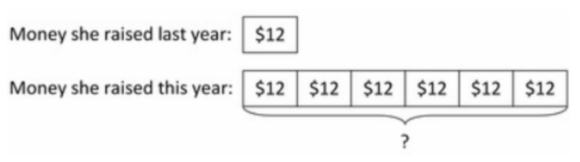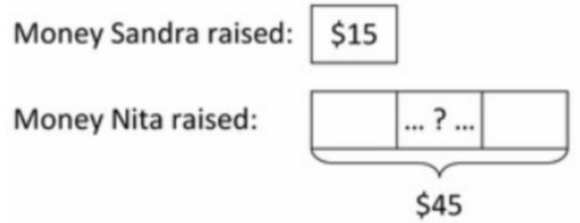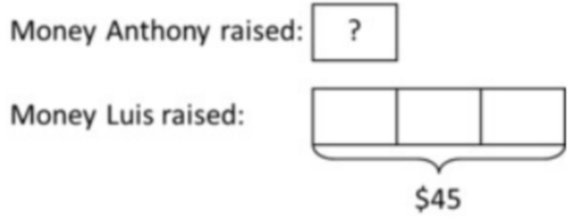• 09/08/13   |   Adjusted: 08/01/18   |   1 file# Comparing Money Raised

Author: Illustrative Mathematics

• Description
• Files

Mathematically:

• Addresses standards: 4.OA.A.14.OA.A.2, and MP.4
• Highlights multiplicative comparison, an important development in multiplicative thinking beginning in grade 4 (4.OA.A.1, 4.OA.A.2)
• Builds meaning for multiplication equations through word problems 4.OA.A); shows how a multiplication equation models a situation (MP.4)
• Uses the same fact family in parts (b) and (c) to highlight different roles of the unknown factors

In the classroom:

• Provides a context that can be acted out in an engaging way
• Allows opportunity for presentation/demonstration of student work (e.g. poster presentation with number strips)

This task was designed to include specific features that support access for all students and align to best practice for English Language Learner (ELL) instruction. Go here to learn more about the research behind these supports. This lesson aligns to ELL best practice in the following ways:

• Provides opportunities for students to practice and refine their use of mathematical language.
• Allows for whole class, small group, and paired discussion for the purpose of practicing with mathematical concepts and language.
• Includes a mathematical routine that reflects best practices to supporting ELLs in accessing mathematical concepts.
• Provides students with support in negotiating written word problems through multiple reads and/or multi-modal interactions with the problem.

• Making the Shifts

How does this task exemplify the instructional Shifts required by CCSSM?

 Focus Belongs to the major work of fourth grade Coherence Builds on previous understanding of multiplication as equal groups to multiplication as times-as-much Rigor Conceptual Understanding: primary in this taskProcedural Skill and Fluency: not targeted in this taskApplication: primary in this task

a. Helen raised $12 for the food bank last year and she raised six times as much money this year. How much money did she raise this year? b. Sandra raised \$15 for the PTA and Nita raised \$45. How many times as much money did Nita raise as compared to Sandra? c. Luis raised$45 for the animal shelter, which was 3 times as much money as Anthony raised. How much money did Anthony raise?

• Illustrative Mathematics Commentary and Solution

Commentary:

The purpose of this task is for students to solve three comparisons problems that are related by their context but are structurally different. Multiplicative comparison is purposefully excluded from third grade (see 3.OA.A.3 and 3.MD.A.2), making this task appropriate for fourth but not third grade.

In these multiplicative comparison problems, one factor and the product are amounts of money and the other factor represents the number of times bigger one amount is than the other. Sometimes this second factor is called a "scale factor." In part (a), the larger amount (which is the product) is unknown, while in part (b) the scale factor is unknown and in part (c) the smaller amount of money is unknown. Students will study multiplicative comparison problems involving scale factors that are fractions in fifth grade; see 5.NF.B.5. Note that in fourth grade, scale factors must always be bigger than 1, so students often think that "multiplying makes bigger"; however in fifth grade they will see that when the scale factor is less than 1, the product wil actually be smaller than the initial quantity.

Note that the numbers in parts (b) and (c) are related by the fact family $3×15=45$. This allows for a classroom discussion about the different interpretations of the factors in a mutiplicative comparison context.

Solution One: Tape Diagram

a. She raised six times as much money (as shown in the diagram) so she raised $6×12=72$.Helen raised \$$72 this year. b. ?×15=45 is equivalent to 45÷15=?Nita raised 3 times as much as Sandra. c. 3×?=45 is equivalent to 45÷3=?Anthony raised \$$15$. Solution 2: Writing multiplication equations for division problems a. Helen raised$6×\$12$ this year, so she raised \$$72 this year. b. This is a "Number of Groups Unknown" problem. We can represent the question as ?×15=45 or 45÷15=? So Nita raised 3 times as much money as Sandra. c. This is a "Group Size Unknown" problem. We can represent the question as 3×?=45 or 45÷3=?. So Anthony raised \$$15\$.